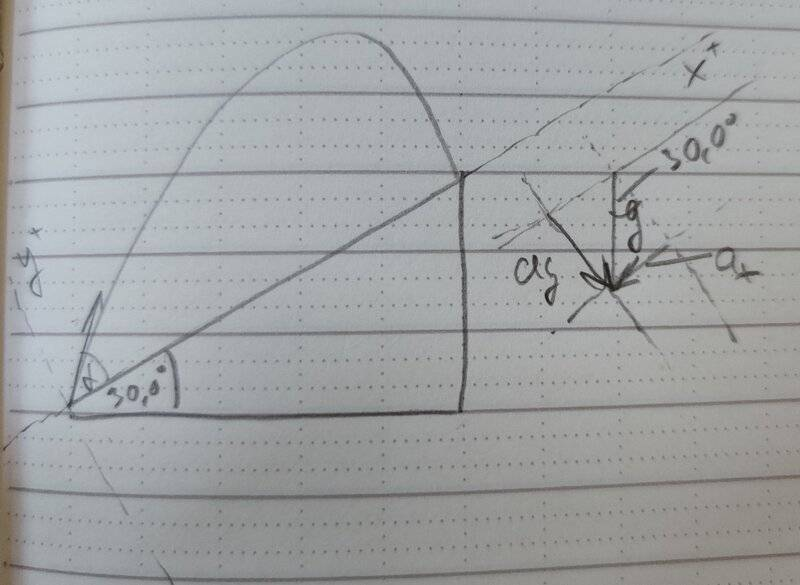# Projectile Motion on an Incline

Argonaut
Homework Statement:
An archer on ground that has a constant upward slope of 30.0° aims at a target 60.0 m farther up the incline. The arrow in the bow and the bull's-eye at the center of the target are each 1.50 m above the ground. The initial velocity of the arrow just after it leaves the bow has magnitude 32.0 m/s. At what angle above the horizontal should the archer aim to hit the bull's eye? If there are two such angles, calculate the smaller of the two. You might have to solve the equation for the angle by iteration that is, by trial and error. How does the angle compare to that required when the ground is level, with 0 slope? [Sears and Zemansky's University Physics. 13th edition. Exercise 3.87]
Relevant Equations:
Equations of 2D position, velocity, and acceleration with constant accelerationI worked myself into a trigonometry rut. I've tried two approaches, first by not changing the frame of reference, and second by taking the incline as the horizontal x axis. Here is my second attempt:

Take the incline as the horizontal. Then the coordinates of target T are:

\begin{align} x_T &= 60.0~\rm{m} \\ y_T &=0 \end{align}

The magnitude of the initial velocity of arrow A and the components of its acceleration are:

\begin{align} v_0 &= 32.0~\rm{m/s} \\ a_x &= -g*\sin{30.0^{\circ}} \\ a_y &= -g*\cos{30.0^{\circ}} \end{align}

Let ##\alpha## be the angle of the initial velocity of the arrow above the incline. Then the components of the initial velocity are:

\begin{align} v_{0x}&=v_0\cos{\alpha} \\ v_{0y}&=v_0\sin{\alpha} \end{align}

The x and y coordinates of the position of the arrow as a function of time are:

\begin{align} x_A &= v_{0x}t + \frac{1}{2}a_xt^2\\ y_A &= v_{0y}t + \frac{1}{2}a_yt^2 \end{align}

At time t when the arrow hits the target, ##x_A = x_T## and ##y_A = y_T##. Which means that I have two unknown values ##\alpha## and ## t##.

Since ##y_A = y_T = 0##, I use it to express t as a function of ##\alpha##.

\begin{align} 0 &= v_0\sin{\alpha}~t+\frac{1}{2}a_yt^2 \\ t&=-\frac{2v_0\sin{\alpha}}{a_y} \end{align}

Then substituting t in the ##x_A## equation, and doing some algebraic manipulation I get:

\begin{align} x_T &= \sin^2{\alpha}~\frac{2v_0^2a_x}{a_y^2}-\sin{\alpha}\cos{\alpha}~\frac{2v_0^2}{a_y} \end{align}

For the sake of clarity, let constants ##A=\frac{2v_0^2}{a_y}## and ##B={a_x}/{a_y}##. Then I have the following equation, where ##\alpha## is the only unknown:

\begin{align} \frac{x_T}{A}&=B\sin^2{\alpha} - \sin{\alpha}\cos{\alpha} \\ &=\sin{\alpha}(B\sin{\alpha} - \cos{\alpha}) \end{align}

And this is where I'm stuck. I've tried various trigonometric identities, double and half-angle formulas, but I couldn't make them work.

(edit: LaTex formatting mistakes)

Last edited:

Homework Helper
Gold Member
2022 Award
\begin{align} \frac{x_T}{A}=B\sin^2{\alpha} - \sin{\alpha}\cos{\alpha} \\ \frac{x_T}{A}=\sin{\alpha}(B\sin{\alpha} - \cos{\alpha}) \end{align}
Perhaps the simplest approach is to aim for a quadratic in ##\sin^2 \alpha##:
$$\frac{x_T}{A} = B\sin^2 \alpha - \sin\alpha \sqrt{1 - \sin^2 \alpha}$$$$\sin\alpha \sqrt{1 - \sin^2 \alpha} = B\sin^2 \alpha - \frac{x_T}{A}$$Now square that equation.

That said, I'm sure there must be a simpler approach. Making gravity two dimensional doesn't look like a good idea to me!

•topsquark and Argonaut
Argonaut
Now square that equation.

That said, I'm sure there must be a simpler approach. Making gravity two dimensional doesn't look like a good idea to me!

Ah right! I feel so silly because I considered that approach but didn't follow through, but now I see that it's essentially a quadratic equation. Thank you(My first attempt didn't make gravity two dimensional, but it led to an equation equally messy, and I didn't perceive it as any simpler. If there's a simpler approach, I'd love to hear about it!)

Homework Helper
Gold Member
2022 Award
Ah right! I feel so silly because I considered that approach but didn't follow through, but now I see that it's essentially a quadratic equation. Thank you(My first attempt didn't make gravity two dimensional, but it led to an equation equally messy, and I didn't perceive it as any simpler. If there's a simpler approach, I'd love to hear about it!)
Okay, it will have to be later today.

•Argonaut
Argonaut
I've solved the quadratic equation in the meantime and the answer is that that the larger angle is 70.7° above horizontal and the smaller is 49.3° which agrees with the answer in the back of the book.

Homework Helper
Gold Member
2022 Award
First, you can express the trajectory of a projectile as a parabolic function:
$$y = y_0 + x\tan \theta - \frac{g}{2v^2}(\sec^2 \theta)x^2$$If you haven't already done so, it's a useful exercise to derive that. Note that you can get everything in terms of ##\tan \theta##, which is often useful:
$$y = y_0 + x\tan \theta - \frac{g}{2v^2}(1 + \tan^2 \theta)x^2$$In this problem, you want the point where this parabola intersects the incline, which is described by:$$y = (\tan \alpha)x$$where ##\alpha## is the angle of the incline. At the point of intersection, we have:
$$\tan \alpha = \tan \theta - \frac{g}{2v^2}(1 + \tan^2 \theta)x_T$$And that's a quadratic in ##\tan \theta##.

•Argonaut and topsquark
Argonaut
First, you can express the trajectory of a projectile as a parabolic function:
$$y = y_0 + x\tan \theta - \frac{g}{2v^2}(\sec^2 \theta)x^2$$If you haven't already done so, it's a useful exercise to derive that. Note that you can get everything in terms of ##\tan \theta##, which is often useful:
$$y = y_0 + x\tan \theta - \frac{g}{2v^2}(1 + \tan^2 \theta)x^2$$In this problem, you want the point where this parabola intersects the incline, which is described by:$$y = (\tan \alpha)x$$where ##\alpha## is the angle of the incline. At the point of intersection, we have:
$$\tan \alpha = \tan \theta - \frac{g}{2v^2}(1 + \tan^2 \theta)x_T$$And that's a quadratic in ##\tan \theta##.
Thanks for the insight and your time - I appreciate it.

•PeroK